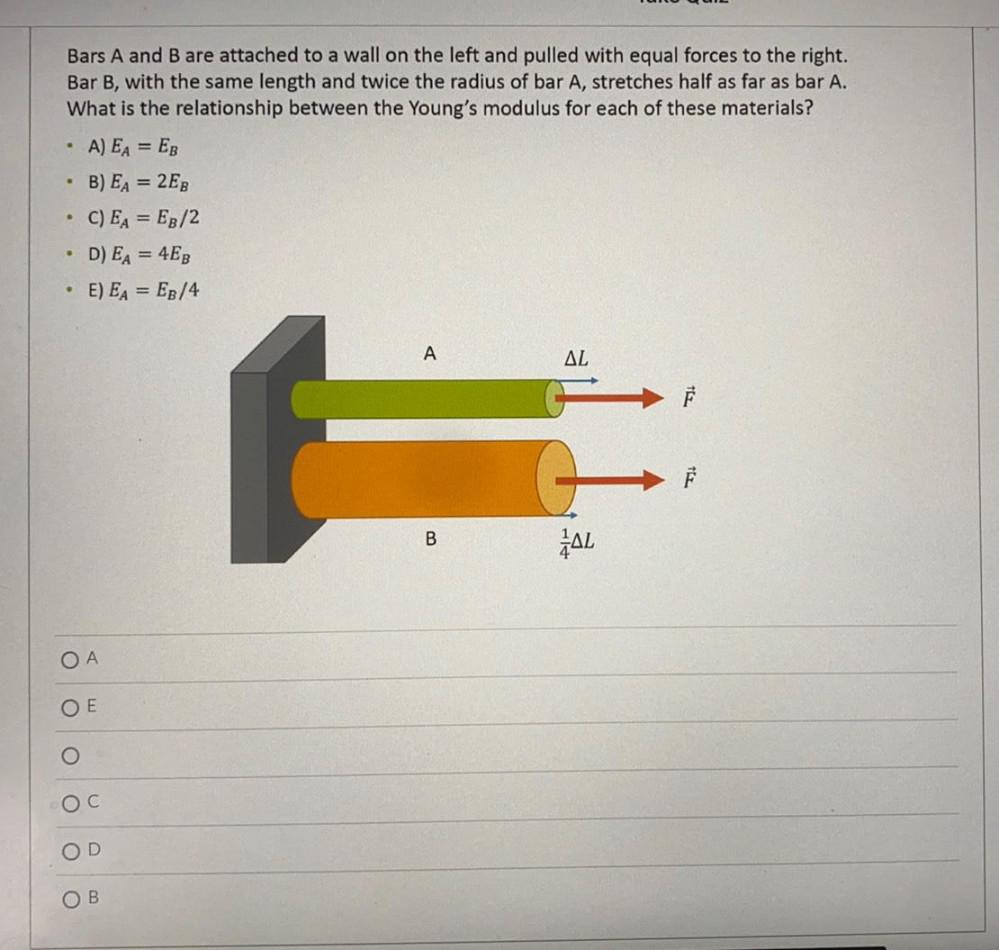Question:

# Bars A and B are attached to a wall on the left and pulled with equal forces to the right. Bar B, with the same length and twiceBars A and B are attached to a wall on the left and pulled with equal forces to the right. Bar B, with the same length and twice the radius of bar A, stretches half as far as bar A. What is the relationship between the Young's modulus for each of these materials? A) EA = EB B) EA = 2EB C) EA = EB/2 D) EA = 4EB E) EA = EB/4 . . . A AL TEL B LAL OA OE O OB